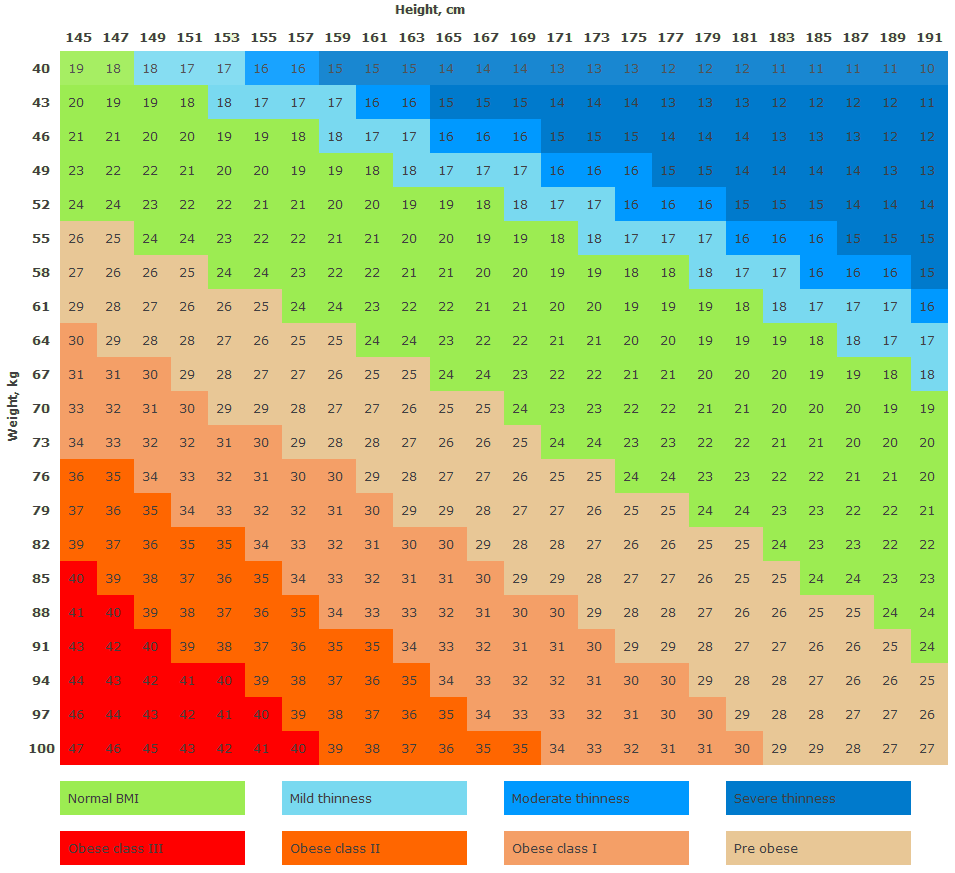## BMI chart for men and women (imperial)

These Body Mass Index (BMI) tables are produced using World Health Organization BMI classification. According to this classification:

BMI
Normal weight 19-24.9
Overweight 25-29.9
Obesity level I 30-34.9
Obesity level II 35-39.9
Obesity level III ≥ 40

BMI chart for men and BMI chart for women are the same and calculated by this BMI formula. Check this is imperial body mass index table (metric chart can be found here) or use this BMI Calculator to check your body mass index.Imperial body mass index chart

## BMI formula

Here is BMI formula if you don’t want to use our BMI Calculator and calculate body mass index manually.

It is very simple:

• Imperial or English BMI formula:
BMI = [weight_in_pounds / (height_in_inches * height_in_inches)] *703
Example: weight = 145lbs, height = 6 ft 1 in (ie 73 inches) and BMI = [ 145 / (73 * 73) ] * 703 = 19.13
• Metric BMI formula:
BMI = weight_in_kilograms / (height_in_meters * height_in_meters)
Example: weight = 70kg, height = 1.80m and BMI = 70 / (1.8 * 1.8) = 21.6

Check BMI charts into which category your BMI falls.

## BMI chart for men and women (metric)

These Body Mass Index (BMI) tables are produced using World Health Organization BMI classification. According to this classification:

BMI
Normal weight 19-24.9
Overweight 25-29.9
Obesity level I 30-34.9
Obesity level II 35-39.9
Obesity level III ≥ 40

BMI chart for men and BMI chart for women are the same and calculated by this BMI formula. Check this is metric body mass index table (imperial chart can be found here) or use this BMI Calculator to check your body mass index.Metric body mass index chart